Study Materials

# NCERT Solutions for Class 9th Mathematics

Page 2 of 9

## Chapter 13. Surface Areas and Volumes

### Exercise 13.2

EXERCISE 13.2

Assume that π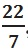​  , unless stated otherwise.

Q1. The curved surface area of a right circular cylinder of height 14 cm is 88 cm2. Find the diameter of the base of the cylinder.

Solution:

H = 14cm,    curved surface area of cylinder = 88cm2

Curved surface area of cylinder = 88cm2

2 ×  × r × h = 88cm2

2 ×  × r × 14 = 88cm2

88r = 88

r = 88/88

⇒ r = 1cm

D = 2r     2 ×1 = 2cm

Q2. It is required to make a closed cylindrical tank of height 1 m and base diameter 140 cm from a metal sheet. How many square metres of the sheet are required for the same?

Solution:

D = 140cm    r = 70cm = 0.7m,        H = 1m

Total surface area of cylinder = 2πr(r + h)

2 × × 0.7 (0.7 + 1)

2 × 22 × 0.1 × 1.7

7.48m2

Hence, 7.48m2 metal sheet required to make closed cylindrical tank.

Q3. A metal pipe is 77 cm long. The inner diameter of a cross section is 4 cm, the outer diameter being 4.4 cm (see Fig. 13.11). Find its

(i) Inner curved surface area,(ii) Outer curved surface area,

(iii) Total surface area.

Solution:

H = 77cm,       H = 77cm

D = 4cm,         D = 4.4cm

R = 2cm,         R = 2.2cm

(i) interior curved surface area = 2πrh

2 ×   × 2 ×77

88 × 11

968 cm2

(ii) exterior curved surface area = 2πrh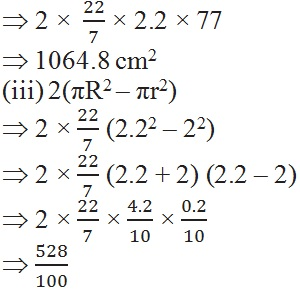5.28 cm2

Total surface area = interior surface area + exterior surface area + ring surface area

1064.8 + 9.68 + 5.28 cm2

2038.08 cm2

Q4. The diameter of a roller is 84 cm and its length is 120 cm. It takes 500 complete revolutions to move once over to level a playground. Find the area of the playground in m2.

Solution:

D = 84cm     r = 42cm,        H = 120cm

Curved surface area of cylinder = 2πrh

2 ×  × 42 × 120

44 × 6 × 120

31680 cm2

Total area of playground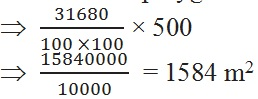Q5. A cylindrical pillar is 50 cm in diameter and 3.5 m in height. Find the cost of painting the curved surface of the pillar at the rate of 12.50 per m2.

Solution:

D = 50 cm ,    R = 0.25m,     H = 3.5m

Curved surface area of cylinder = 2πrh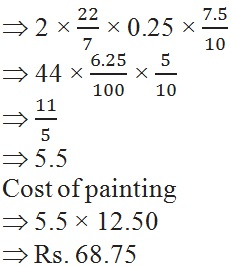Q6. Curved surface area of a right circular cylinder is 4.4 m2. If the radius of the base of the cylinder is 0.7 m, find its height.

Solution:

Curved surface area of cylinder = 4.4 m2 ,    radius = 0.7 m

Let the height of the cylinder be = h

2πrh = 4.4Q7. The inner diameter of a circular well is 3.5 m. It is 10 m deep. Find

(i) its inner curved surface area,

(ii) the cost of plastering this curved surface at the rate of 40 per m2.

Solution:

Inner diameter of circular well = 3.5 m   r = 1.75 m

Depth of the well = 10 m

(i) inner surface area of the well = 2πrh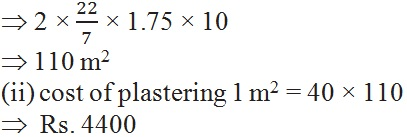Q8. In a hot water heating system, there is a cylindrical pipe of length 28 m and diameter 5 cm. Find the total radiating surface in the system.

Solution:

The length of the pipe = 28 m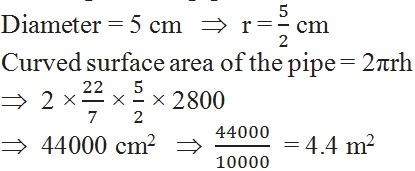Q9. Find

(i) the lateral or curved surface area of a closed cylindrical petrol storage tank that is 4.2 m in diameter and 4.5 m high.

(ii) how much steel was actually used, if of the steel actually used was wasted in making the tank.

Solution:

(i) diameter of the cylindrical petrol tank = 4.2 m

Radius of the tank = 2.1m ,    height = 4.5 m

Curved surface area of cylinder = 2πrhQ10. In Fig. 13.12, you see the frame of a lampshade. It is to be covered with a decorative cloth. The frame has a base diameter of 20 cm and height of 30 cm. A margin of 2.5 cm is to be given for folding it over the top and bottom of the frame. Find how much cloth is required for covering the lampshade.

Solution:

Height of the folding = 2.5 cm

Height of the frame = 30 cm

Diameter = 20 cm    radius = 10 cm

Now cloth required for covering for lampshade

= C.S.A of top + C.S.A of middle + C.S.A of bottom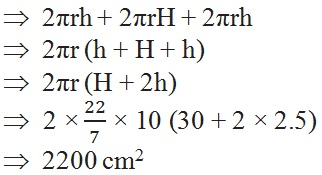Q11. The students of a Vidyalaya were asked to participate in a competition for making and decorating penholders in the shape of a cylinder with a base, using cardboard. Each penholder was to be of radius 3 cm and height 10.5 cm. The Vidyalaya was to supply the competitors with cardboard. If there were 35 competitors, how much cardboard was required to be bought for the competition?

Solution:

Radius of pen holder = 3 cm

Height of pen holder = 10.5 cm

Cardboard required for pen holder = CSA of pen holder + area of circular base

2πrh + πr2

πr(2h + r)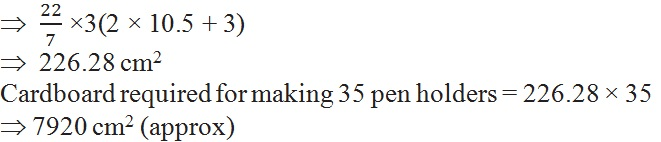Page 2 of 9

Chapter Contents: